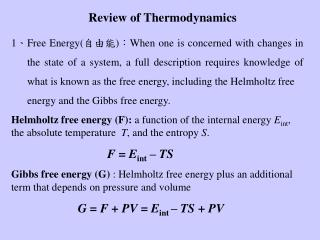DownloadDownload PresentationReview of Thermodynamics

# Review of Thermodynamics

Download Presentation## Review of Thermodynamics

- - - - - - - - - - - - - - - - - - - - - - - - - - - E N D - - - - - - - - - - - - - - - - - - - - - - - - - - -
##### Presentation Transcript

1. Review of Thermodynamics 1、Free Energy(自由能)：When one is concerned with changes in the state of a system, a full description requires knowledge of what is known as the free energy, including the Helmholtz free energy and the Gibbs free energy. Helmholtz free energy (F): a function of the internal energy Eint, the absolute temperature T, and the entropy S. F = Eint – TS Gibbs free energy (G) : Helmholtz free energy plus an additional term that depends on pressure and volume G = F + PV = Eint – TS + PV

2. 2、Equilibrium conditions：the general rule is that a system can spontaneously alter its status if the change results in a lowering of the free energy. Therefore the equilibrium condition is that the free energy of the system is minimized. For a mechanically isolated system at constant temperature (no work) F 0 ( = holds at equilibrium ) For a system kept at constant temperature and pressure G 0 ( = holds at equilibrium )

3. Thermodynamic Potentials: Internal Energy (Eint), Enthalpy (H), Helmholtz Free Energy (F), Gibbs Free energy (G) H = Eint +PV, F = Eint-TS, G = Eint-TS+PV Maxwell Relations:

4. V1 V2 3、Example of the principle of minimizing free energy. Consider an isolated ideal gas system consisting of two compartments of volume V1 and V2, respectively. The boundary between these two compartments is free to moveFind the equilibrium condition. Sol： For an isolated system, the equilibrium condition is -P1 -P2 Equilibrium condition：P1=P2

5. Ekin = 0 Epot> 0 Ekin> 0 Epot= 0 Ekin= 0 Epot> 0 Ekin> 0 Epot= 0 Ekin= 0 Epot> 0 4、Internal Energy Consider two atoms in equilibrium Ekin = 0 Epot = 0 Small perturbation t = 0

6. For a system of N particles equipartition theorem kB = 1.3810-23JK-1：Boltzmann constant 3N degrees of freedom Each degree of freedom: ½ kBT

7. 5、Entropy (S) S = kB ln：number of available states a state involves both the positions and the velocities of all the atoms in the system. Example: Consider a harmonic oscillator; Its Hamiltonian is k m p = momentum q = position phase space Total available area in phase space = W Unit area in p-q phase space due to uncertainty principle is h Number of states:  = W／h p E q For a system of N particles in 3-D space  (E,V,N) = W(E)／h3N E+ E

8. N Example 2：A container is divided into two parts by a barrier that has a small hole in it. The gas molecules are indistinguishable. (b) (a) n1 n2 N = n1 + n2

9. Consider a system that is described by a set of states 1, 2, 3, ...., i,.... with corresponding energies 1, 2, 3, … i,… Let the number of particles in state 1 be n1, in state 2 be n2, …and so on. Total number of particles: Number of different ways: Maximizing entropy with the constraints of particle number and total energy, we get

10. E E2 E E1 configurations ： partition function At equilibrium, the probability to jump from state i to state j is (detail balance)

11. Connection between free energy and partition function Define F = -NkBTlnZ what is F？ if F is the Helmholtz free energy

12. At equilibrium chemical activity Reaction Kinetics fist order second order reaction fluxJc=k2[C] , JA=JB=k1[A][B] chemical potential of a given component A more general reaction is the change in the Gibbs free energy activation energy

13. Water-- Mercedes-Benz model The near-tetrahedral structure of the water molecule provides the basis for the most common crystal structure of ice. Hydrogen bonds in liquid water are perpetually being formed and broken. The hydrophobicity of a non-polar molecule arises due to the reduced entropy of water in the presence of such a non-polar object, which plays an important role in biology. To study water, the MB model in 2-d provides a simplified view of ordering the water configurations in the presence of an ion or a non-polar molecule.

14. Molecular Interactions water-water interaction van der Waals’ term Hydrogen bonding term ion-water interaction

15. water distribution

16. Average number of hydrogen bonds within the first water shell around an ion